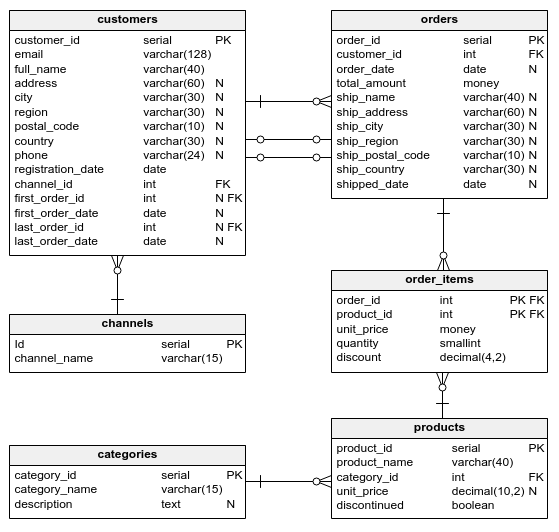Deals Of The Week - hours only!Up to 80% off on all courses and bundles.-Close
Introduction
Conversion rates
4. Global lifetime conversion rate – step 2
Time to first order
Conversion charts
Summary

## Instruction

Great! Now that we know how to find the count of all customers and the count of those who've made a purchase, we can calculate the global lifetime conversion rate:

SELECT ROUND(COUNT(first_order_id) / CAST(COUNT(*) AS float), 2) AS conversion_rate
FROM customers;


We simply divided the number of made-a-purchase customers by the total number of registered customers. Notice that we cast the denominator to a float type using CAST(COUNT(*) AS float). Both the numerator and the denominator are integers, so the database would use integer division by default. The result would be incorrect, so we had to cast one of the values to force float division.

We also used the ROUND(value, number) function, which rounds the given value to the given number of decimal places.

## Exercise

Find the lifetime conversion rate among customers who registered in 2017. Show the result in a column named conversion_rate. Round the result to four decimal places.

### Stuck? Here's a hint!

You need a WHERE clause with the registration_date column.